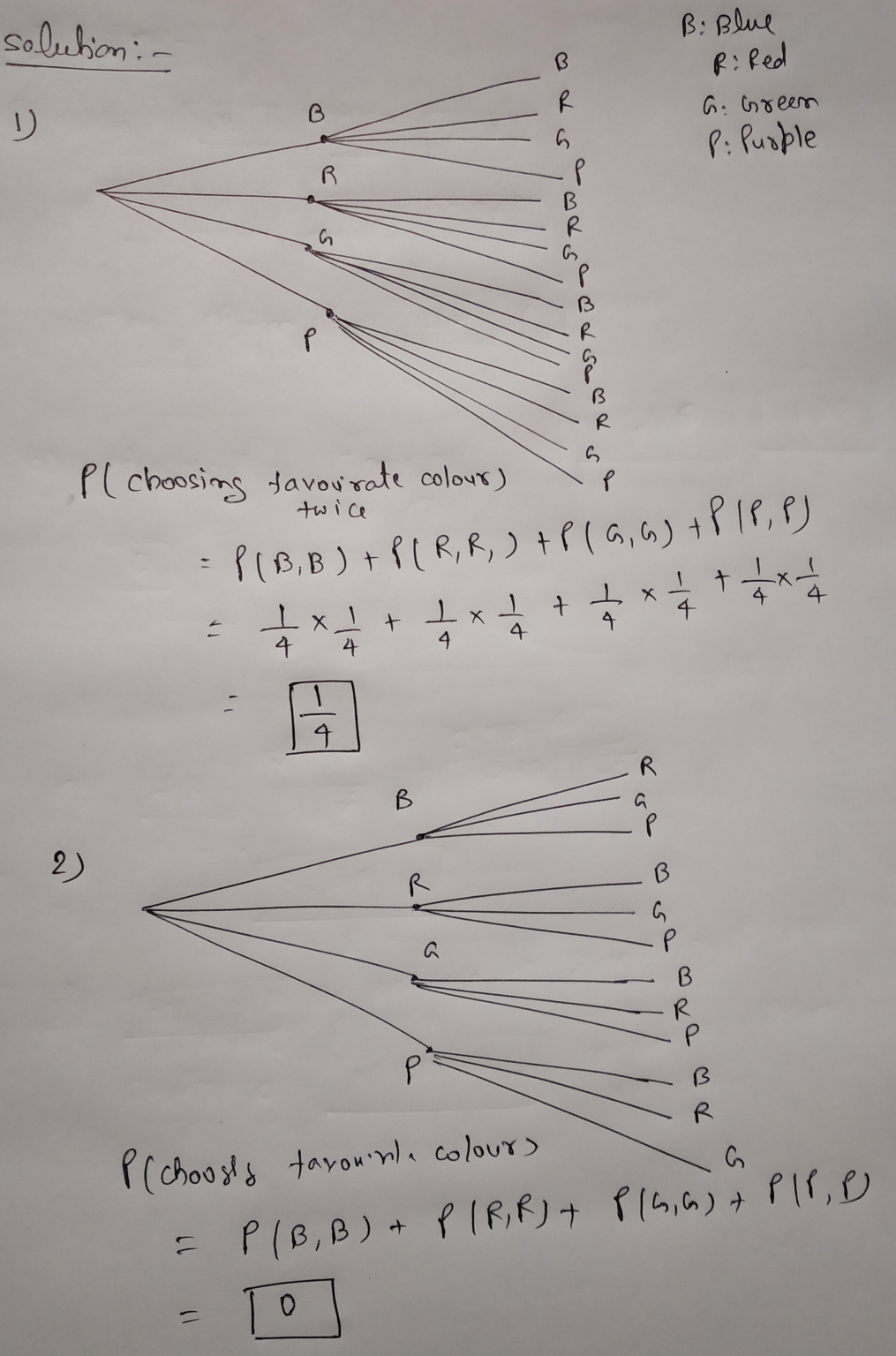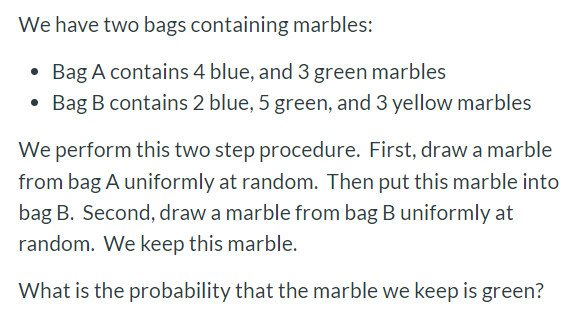# Probabilities - There are 4 marbles in a bag to choose from Blue, Red, Green, and...

Probabilities

- There are 4 marbles in a bag to choose from Blue, Red, Green, and Purple.

You are to select 1 marble, put it back in the bag, and then select the second marble.

Draw a tree diagram for this situation.

What is the probability of choosing your favorite color two times in the row?

- There are 4 marbles in a bag to choose from:

Blue, Red, Green, and Purple.

You are to select 1 marble, keep it, and then select the second marble.

Draw a tree diagram for this situation.

What is the probability of choosing your favorite color two times in the row?#### Earn Coin

Coins can be redeemed for fabulous gifts.

Similar Homework Help Questions
• ### We have two bags containing marbles: • Bag A contains 4 blue, and 3 green marbles...We have two bags containing marbles: • Bag A contains 4 blue, and 3 green marbles • Bag B contains 2 blue, 5 green, and 3 yellow marbles We perform this two step procedure. First, draw a marble from bag A uniformly at random. Then put this marble into bag B. Second, draw a marble from bag B uniformly at random. We keep this marble. What is the probability that the marble we keep is green?

• ### A bag contains 7 red marbles, 2 blue marbles, and 1 green marble

A bag contains 7 red marbles, 2 blue marbles, and 1 green marble. If a marble is selected at random, what is the probability of choosing a marble that is not blue? 7+2+1=10 There are two blue marblesSo is the probability 1/5?

• ### First bag contains 7 blue marbles , 6 green marbles and another bag contains 2 blue...

First bag contains 7 blue marbles , 6 green marbles and another bag contains 2 blue marbles, 4 green marbles and 3 yellow marbles. We draw a random marble from first bag and and place it to the other bag. Then We draw a marble from the second bag randomly and keep this one. Calculate the probability that the marble we pick is green.

• ### a bag contains 7 red marbles, 8 blue marbles and 4 green marbles

a bag contains 7 red marbles, 8 blue marbles and 4 green marbles. what is the probablity of choosing a blue marble?

• ### if a bag contains 8 red marbles, 6 blue marbles and 9 green marbles, what is the probability of choosing a blue marble

if a bag contains 8 red marbles, 6 blue marbles and 9 green marbles, what is the probability of choosing a blue marble??

• ### There are 3 green marbles and 5 blue marbles in a bag and 4 red marbles....

There are 3 green marbles and 5 blue marbles in a bag and 4 red marbles. Two marbles are drawn from the bag at random without replacement. Find the probability that both marbles are blue. Find the probability that the first is blue and the second green.

• ### A bag contains 7 blue marbles, 5 red marbles, and 9 green marbles

A bag contains 7 blue marbles, 5 red marbles, and 9 green marbles. A marble is taken at random from the bag. What is the probability that the marble is blue or red? Please type in your answer as a fraction.

• ### A bag contains 7 red marbles, 2 blue marbles, and 1 green marble

A bag contains 7 red marbles, 2 blue marbles, and 1 green marble. If a marble is selected at random, what is the probability of choosing a marble that is not blue?I have seen so many different answers on this site to this question and am not sure. I thought it would be 7 red +1 green gives 8/10=4/5.Our choices for the answer is 4/5,1/5, 5/4, 8

• ### Suppose you have a bag containing two red marbles, two blue marbles, and two white marbles

Suppose you have a bag containing two red marbles, two blue marbles, and two white marbles. You choose two marbles without looking.a. What is the probability that you will choose a red marble and then a blue marble without replacing the red one?b. What is the probability that you will choose two red marbles in a row without replacing the first one?

• ### a bag contains 5 blue, 4 red, 9 white, and 6 green marbles

a bag contains 5 blue, 4 red, 9 white, and 6 green marbles. if a marble is drawn at random and replaced 100 times, how many would you expect to draw a green marble?